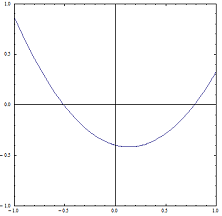Manfred BörgensMathematical Problems problem listprevious problem      next problem main pagedeutsche Version

Parabola in a Square

We define the quadratic polynomial  f  by  f(x) = x2 + b.x + c  with  b,c  R .

Which constants  b,c  suit the constraint  f: [-1,1] → [-1,1] ?

Hence, we are looking for all parabolas  f(x) = x2 + b.x + c ,  whose graph for  x  [-1,1]  lies completely in the square  [-1,1]2 .Solution

last update  2019-01-04
previous problem   |    problem list   |    next problem
Manfred Börgens   |    main page